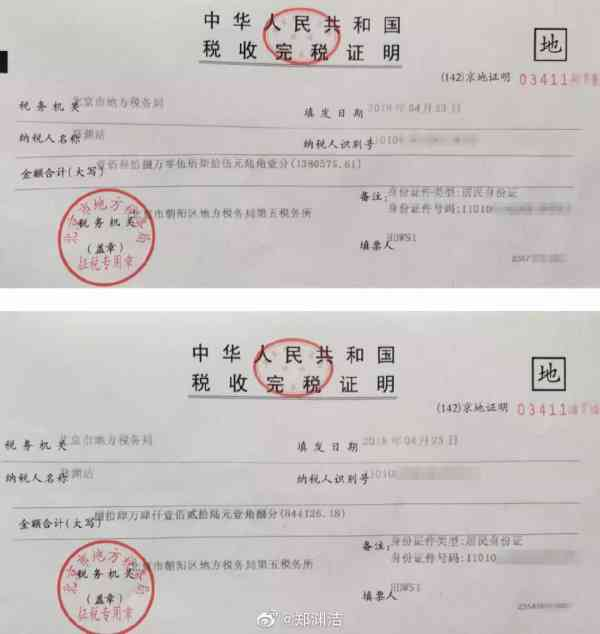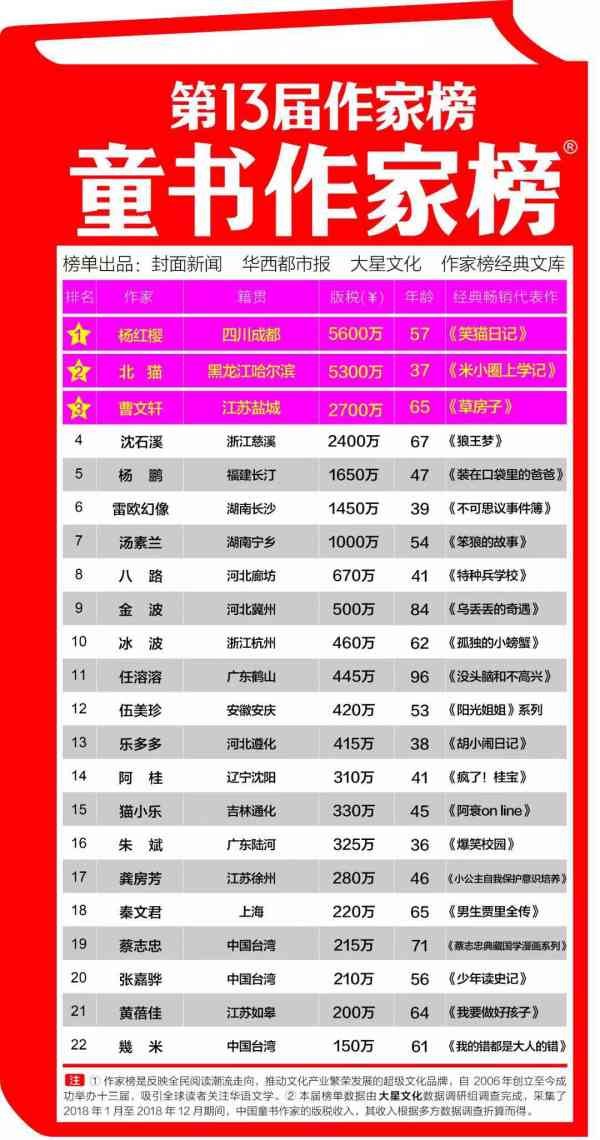var n = new Date(); var y = n.getFullYear(); var m = ((m=n.getMonth()+1)<10?'0'+m:m); var d = ((d=n.getDate())<10?'0'+d:d); var h = ((h=n.getHours())<10?'0'+h:h); var i = ((i=n.getMinutes())<10?'0'+i:i); var w = new Array('日','一','二','三','四','五','六'); document.write(y+"年"+m+"月"+d+"日"+' '+h+':'+i+' '+'星期'+w[n.getDay()]);

# 郑渊洁回应未登“童书作家榜”，炮轰作家进校园售书

（原题为《郑渊洁回应未登“童书作家榜” ，“当我获悉中国作家榜制榜方首次将童书作家销售排名，晒出两张税单面额分别为1380575.61元和844126.18元。0.1307s , 18150.59375 kb